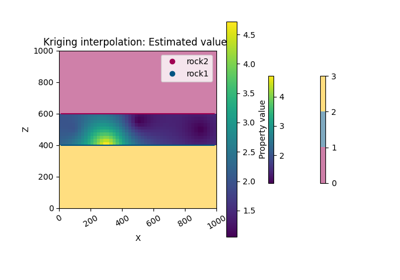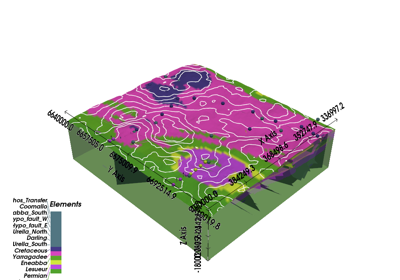# gempy.core.interpolator.InterpolatorModel¶

class gempy.core.interpolator.InterpolatorModel(surface_points: gempy.core.data_modules.geometric_data.SurfacePoints, orientations: gempy.core.data_modules.geometric_data.Orientations, grid: gempy.core.data.Grid, surfaces: gempy.core.data.Surfaces, series, faults: gempy.core.data_modules.stack.Faults, additional_data: gempy.core.data.AdditionalData, **kwargs)[source]

Child class of Interpolator which set the shared variables and compiles the theano graph to compute the geological model, i.e. lithologies.

compute_weights_ctrl

[s0] List that controls what parts of the graph for each series have to be recomputed

Type

list[bool]

compute_scalar_ctrl
Type

list[bool]

compute_block_ctrl
Type

list[bool]

Interpolator Doc
(inserted)

Class that act as:

1. linker between the data objects and the theano graph

2. container of theano graphs + shared variables

3. container of theano function

Args:

surface_points (SurfacePoints): [s0] Data child with specific methods to manipulate interface data. It is initialize without arguments to giveflexibility to the origin of the data.

orientations (Orientations): [s1] Data child with specific methods to manipulate orientation data. It is initialize without arguments to giveflexibility to the origin of the data.

grid (Grid): [s2] Class to generate grids.

surfaces (Surfaces): [s3] Class that contains the surfaces of the model and the values of each of them.

series (Series): [s4] Class that contains the functionality and attributes related to the series. Notice that series does not onlyrefers to stratigraphic series but to any set of surfaces which will be interpolated together (comfortably).

faults (Faults): [s5] Class that encapsulate faulting related content. Mainly, which surfaces/surfaces are faults. The fault network—i.e. which faults offset other faults—and fault types—finite vs infinite.

additional_data (AdditionalData): [s6] Container class that encapsulate Structure, KrigingParameters, Options and rescaling parameters

kwargs:

• compile_theano: if true, the function is compile at the creation of the class

Attributes:

surface_points (SurfacePoints)

orientations (Orientations)

grid (Grid)

surfaces (Surfaces)

faults (Faults)

dtype ([‘float32’, ‘float64’]): float precision

theano_graph: theano graph object with the properties from AdditionalData -> Options

theano function: python function to call the theano code

Examples using InterpolatorModelMethods

 __init__(surface_points, orientations, grid, …) Initialize self. calculate_V(centered_grid) calculate_tz(centered_grid) compile_th_fn_geo([inplace, debug, grid]) Compile and create the theano function which can be evaluated to compute the geological models create_theano_graph([additional_data, …]) Create the graph accordingly to the options in the AdditionalData object get_python_input_block([append_control, …]) Get values from the data objects used during the interpolation: Modify all theano shared matrices to the right size according to the structure data. Modify the theano shared weights vector according to the structure. Print many of the theano shared variables remove_series_without_data() Method to reset to the initial state all the recompute ctrl. After calling this method next time set_all_shared_parameters([reset_ctrl]) Set all theano shared parameters required for the computation of lithology Initialize the ctrl vectors to the number of series size. Initialize all the theano shared variables where we store the final results of the interpolation. Initialize all the theano shared variables where we store the final results of the interpolation except the kriging weights vector. set_theano_graph(th_graph) Attach an already create theano graph. set_theano_shared_Vs_kernel([V]) set_theano_shared_fault_relation() Set all theano shared variables wich controls the faults behaviour set_theano_shared_gravity([tz, pos_density]) set_theano_shared_grid([grid]) Set theano shared variable which controls if a series is fault or not Set theano shared variable which controls if a fault is finite or not set_theano_shared_kriging() Set to the theano_graph attribute the shared variables of kriging values from the linked set_theano_shared_l0_l1() Set the theano shared variables that are looped for each series. set_theano_shared_magnetic_cts(incl, decl[, …]) param B_ext External magnetic field in [T], in magnetic surveys this is the geomagnetic field - varies temporaly set_theano_shared_magnetics([V, …]) set_theano_shared_nuggets() Set the theano variables which control the masking patterns according to the uncomformity relation set_theano_shared_pos_density(pos_density) set_theano_shared_pos_magnetics(pos_magnetics) Set all theano shared variables that control all the series interactions with each other set_theano_shared_structure([reset_ctrl]) Set all theano shared variable dependent on Structure. set_theano_shared_structure_surfaces() Set to the theano_graph attribute the shared variables of structure from the linked set_theano_shared_topology() set_theano_shared_tz_kernel([tz]) Set the theano component tz to each voxel Set the theano shared weights and [s0] Set the theano shared variables that are looped for each series
__init__(surface_points: gempy.core.data_modules.geometric_data.SurfacePoints, orientations: gempy.core.data_modules.geometric_data.Orientations, grid: gempy.core.data.Grid, surfaces: gempy.core.data.Surfaces, series, faults: gempy.core.data_modules.stack.Faults, additional_data: gempy.core.data.AdditionalData, **kwargs)[source]

Initialize self. See help(type(self)) for accurate signature.

reset_flow_control_initial_results(reset_weights=True, reset_scalar=True, reset_block=True)[source]
Method to reset to the initial state all the recompute ctrl. After calling this method next time

gp.compute_model is called, everything will be computed. Panic bottom.

Parameters
• reset_weights (bool) –

• reset_scalar (bool) –

• reset_block (bool) –

Returns

True

set_flow_control()[source]

Initialize the ctrl vectors to the number of series size.

Returns

True

set_all_shared_parameters(reset_ctrl=False)[source]

Set all theano shared parameters required for the computation of lithology

Parameters

reset_ctrl (bool) – If true, [s0] Method to reset to the initial state all the recompute ctrl. After calling this method next time gp.compute_model is called, everything will be computed. Panic bottom.

Returns

True

set_theano_shared_structure(reset_ctrl=False)[source]

Set all theano shared variable dependent on Structure.

Parameters

reset_ctrl (bool) – If true, [s0] Method to reset to the initial state all the recompute ctrl. After calling this method next time gp.compute_model is called, everything will be computed. Panic bottom.

Returns

True

set_theano_shared_loop()[source]

Set the theano shared variables that are looped for each series.

set_theano_shared_weights()[source]

Set the theano shared weights and [s0] Set the theano shared variables that are looped for each series

set_theano_shared_is_fault()[source]

Set theano shared variable which controls if a series is fault or not

set_theano_shared_is_finite()[source]

Set theano shared variable which controls if a fault is finite or not

set_theano_shared_onlap_erode()[source]

Set the theano variables which control the masking patterns according to the uncomformity relation

set_theano_shared_faults()[source]

Set all theano shared variables wich controls the faults behaviour

set_theano_shared_relations()[source]

Set all theano shared variables that control all the series interactions with each other

set_initial_results()[source]

Initialize all the theano shared variables where we store the final results of the interpolation. This function must be called always after set_theano_shared_loop

Returns

True

set_initial_results_matrices()[source]

Initialize all the theano shared variables where we store the final results of the interpolation except the kriging weights vector.

Returns

True

modify_results_matrices_pro()[source]

Modify all theano shared matrices to the right size according to the structure data. This method allows to change the size of the results without having the recompute all series

modify_results_weights()[source]

Modify the theano shared weights vector according to the structure.

get_python_input_block(append_control=True, fault_drift=None)[source]
Get values from the data objects used during the interpolation:
• dip positions XYZ

• dip angles

• azimuth

• polarity

• surface_points coordinates XYZ

Parameters
• append_control (bool) – If true append the ctrl vectors to the input list

• fault_drift (Optional[np.array]) – matrix with per computed faults to drift the model

Returns

list of arrays with all the input parameters to the theano function

Return type

list

print_theano_shared()[source]

Print many of the theano shared variables

compile_th_fn_geo(inplace=False, debug=True, grid: Optional[Union[str, numpy.ndarray]] = None)[source]

Compile and create the theano function which can be evaluated to compute the geological models

Parameters
• inplace (bool) – If true add the attribute theano.function to the object inplace

• debug (bool) – If true print some of the theano flags

• grid – If None, grid will be passed as variable. If shared or np.ndarray the grid will be treated as constant (if shared the grid will be taken of grid)

Returns

function that computes the whole interpolation

Return type

theano.function